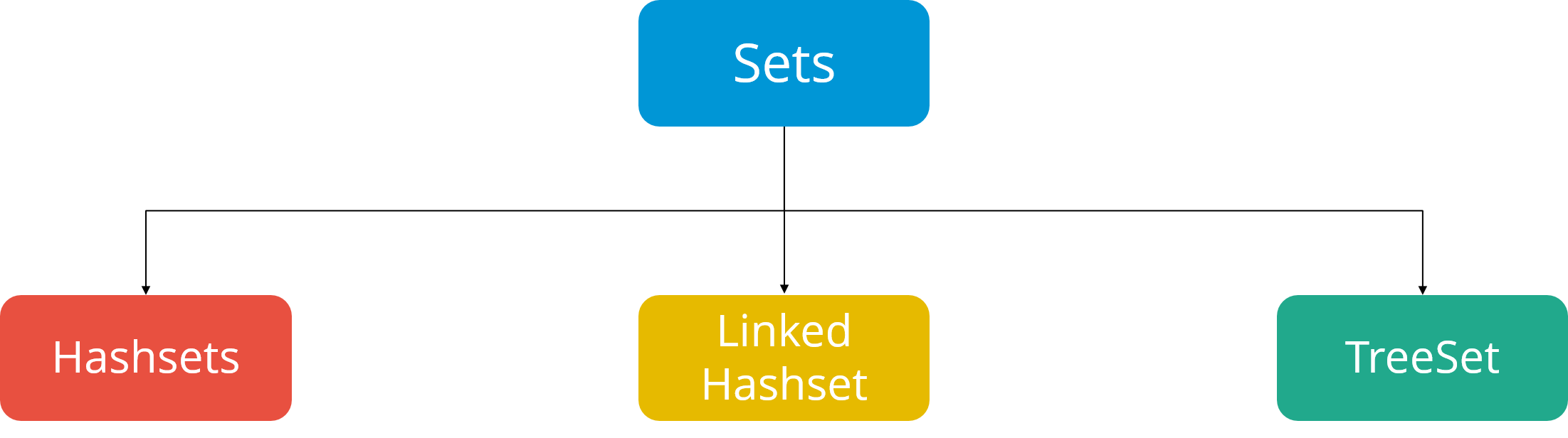# Sets In Java: Know how to work with Java Set Interface

Published on Jul 12,2019 570 ViewsThe Java Collection Framework contains numerous interfaces, one of which is the Set Interface. This article will give you a detailed introduction to Sets In Java. Following are the pointers to be covered in this article:

## Sets In Java

Set be defined as a collection of unordered elements; wherein duplicate values cannot be stored. It extends Collection and thus all methods in the Collection interface are available in the Set interface. It is implemented by HashSet, LinkedHashSet, or the TreeSort.Each of these implementations act differently while iterating the set, mostly with respect to the ordering of the elements, and the time taken for insertion and for accessing the elements.

• Hash Set does not provide any guarantee about the order of the elements while iterating the set.
• LinkedHashSet on the other hand, provides a guarantee about the order of the elements while iterating them.
• TreeSet provides guarantee, but the set is sorted according to the natural order, or by a specific comparator implementation.

## How to create a Set?

The following code defines the method of creating a new set:

`Set<Integer> num = new HashSet<>();`

We have used generics to declare the set of an integer type.

## Set Methods in Java:

We can perform multiple operations on a set such as follows:

The add method inserts an element to the Java collection. In the code below, we insert a set of names.

```Set<String> strName = new HashSet<>();
System.out.println(strName);
```

Output:

[John,  Doe]

### Remove Method

This method removes the specified element from the set.

```import java.util.*;
public class Main{
public static void main(String args[])
{
// Creating an Empty Set
Set<String> set = new HashSet<String>();

// Display the set
System.out.println("Set: " + set);

// Removing the element “Doe” using remove() method
set.remove("Doe");

// Displaying the modified set
System.out.println("Set : "
+ set);
}
}
```

Output:

Set : [John,  Doe]

Set : [John]

### Is Empty Method

This method checks determines whether the set is empty is not. It returns true if the set is empty, and false if otherwise.

```import java.io.*;
import java.util.*;

public class Main {
public static void main(String args[])
{
Set<String> javaSet = new HashSet<String>();

// Adding elements to the Set

// Display the set
System.out.println("Set: " + javaSet);

// Checking whether the set is empty
System.out.println("Empty Set : " + javaSet.isEmpty());

// Clearing the set using the clear() method
javaSet.clear();

// Checking whether the set is empty
System.out.println("Empty Set : " + javaSet.isEmpty());
}
}
```

Output:

Set : [John,  Doe]

Empty Set : false

Empty Set : true

### Size Method

The size() method returns the size of the set, i.e. the number of elements present in the set.

```import java.util.*;
public class Main {
public static void main(String args[])
{
// Creating a set
Set<String> set = new HashSet<String>();

System.out.println("Set: " + set);

// Displaying the size of the sent
System.out.println("Size of the set : " + set.size());
}
}
```

Output:

Set : [John,  Doe]

Size of the set : 2

### Iterating Over A Set

We can iterate over all the elements present in the set by the following method:

```import java.util.*;
import java.util.HashSet;

public class Main {
public static void main(String args[])
{
// Creating a HashSet
HashSet<String> javaSet = new HashSet<String>();

// Displaying the set
System.out.println("HashSet: " + javaSet);

// Creating an iterator
Iterator itr = javaSet.iterator();

// Displaying the values after iteration
System.out.println("Iterator values: ");
while (itr.hasNext()) {
System.out.println(itr.next());
}
}
}
```

Output:

HashSet : [John,  Doe]

Iterator Values:

John

Doe

Searching in  A Set

We use the contains() method to determine whether the set contains a specified element. Returns true if the element is found and false otherwise.

```import java.io.*;
import java.util.HashSet;

public class Main {
public static void main(String args[])
{
// Creating a HashSet
HashSet<String> javaSet = new HashSet<String>();

// Displaying the HashSet
System.out.println("HashSet: " + javaSet);

// Checking for “John” in the set
System.out.println("John in set: " + javaSet.contains("John"));

// Checking for "Hazel" in set
System.out.println("Hazel in set: " + javaSet.contains("Hazel"));
}
}
```

Output:

HashSet : [John,  Doe]

John in set: true

Hazel in set: false

## Basic Operation On Sets in Java

• Union: To add one set to another, we use the Union operation
• Intersection: To retain the common values from both the sets, we use the intersection operation.
• Difference: To remove the values of one set, from the other set, the difference operation is used.

Example

```import java.util.*;
public class Main
{
public static void main(String args[])
{
Set<Integer> d = new HashSet<Integer>();
d.addAll(Arrays.asList(new Integer[] {3, 2, 1, 9, 6, 4, 0}));
Set<Integer> e = new HashSet<Integer>();
e.addAll(Arrays.asList(new Integer[] {3, 1, 9, 5, 2, 0, 7,}));

// Union Operation
Set<Integer> union = new HashSet<Integer>(d);
System.out.println("Union :" + union);

// Intersection Operation
Set<Integer> intersection = new HashSet<Integer>(d);
intersection.retainAll(e);
System.out.println("Intersection :" + intersection);

// Difference Operation
Set<Integer> difference = new HashSet<Integer>(d);
difference.removeAll(e);
System.out.println("Difference :" + difference);
}
}
```

Output:

Union : [0, 1, 2, 3, 4, 5, 6, 7, 9]

Intersection : [0, 1, 2, 3, 9]

Difference : [4, 6]

The methods and the operations mentioned in the method make the set interface elemental and efficient in nature.

Thus we have come to an end of this article on ‘Sets in Java’. If you wish to learn more, check out the Java Training by Edureka, a trusted online learning company. Edureka’s Java J2EE and SOA training and certification course is designed to train you for both core and advanced Java concepts along with various Java frameworks like Hibernate & Spring.

Got a question for us? Please mention it in the comments section of  “sets in java” article and we will get back to you as soon as possible.REGISTER FOR FREE WEBINARThank you for registering Join Edureka Meetup community for 100+ Free Webinars each month